# GPT AI结对编程初体验# 入口

chatGPT火了一段时间，我没试，因为我不想翻墙。因为什么？翻墙违法啊，你不知道？我还在观望之中的时候，GPT-4又来了。火的东西多了，承诺多大令人失望，所以我没有着急。

https://m.weibo.cn/status/4880070764400024?wm=3333_2001&from=10D3193010&sourcetype=weixin&jumpfrom=weibocom

# 贪吃蛇，业务逻辑，修改需求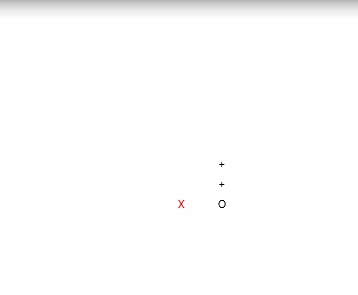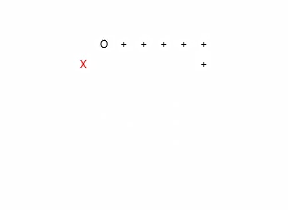# 不能写论文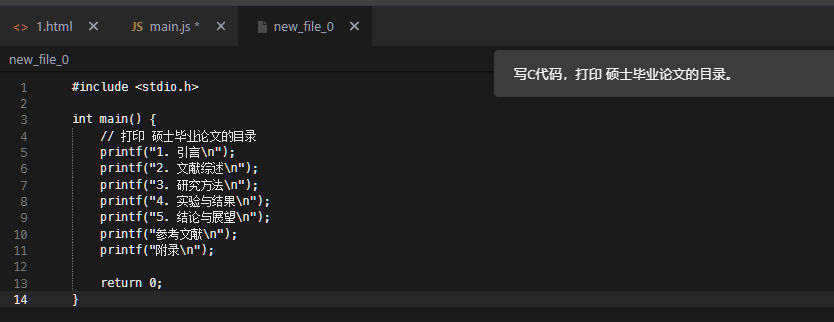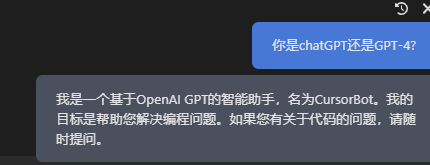# 解读代码

Zhumao贴来一段代码，问，这段代码能读懂不。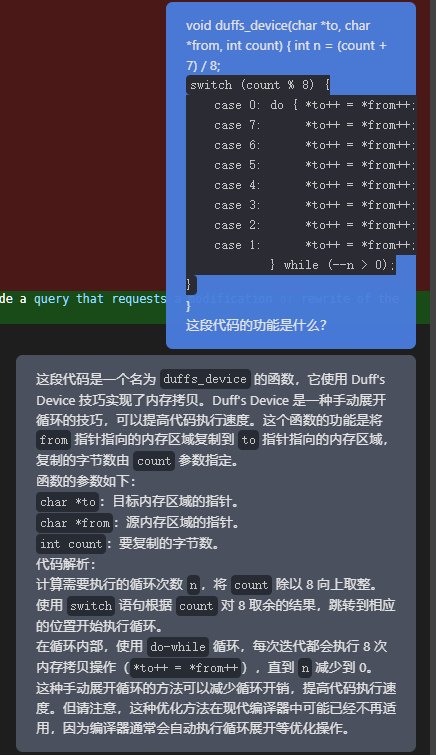# 俄罗斯方块，逐步提示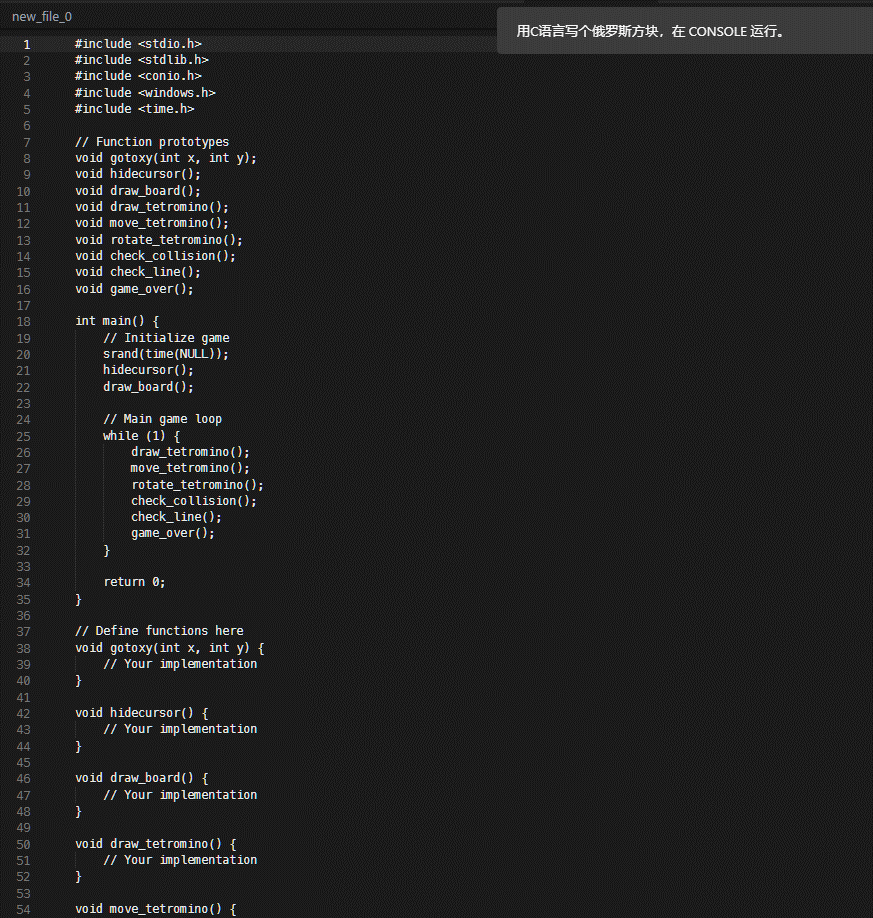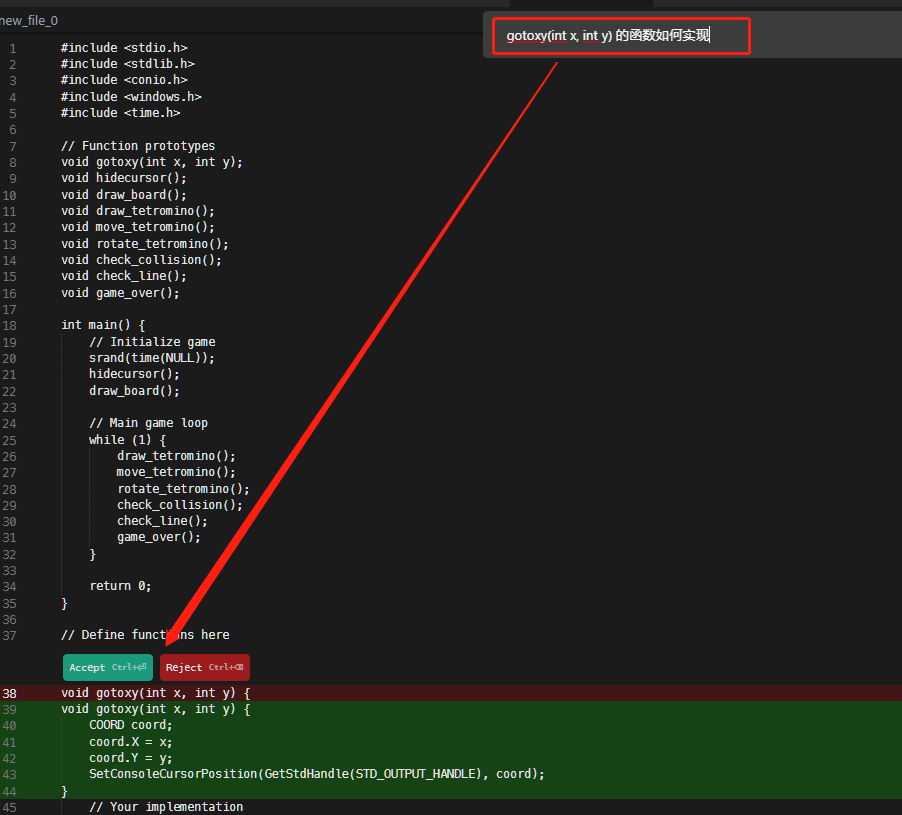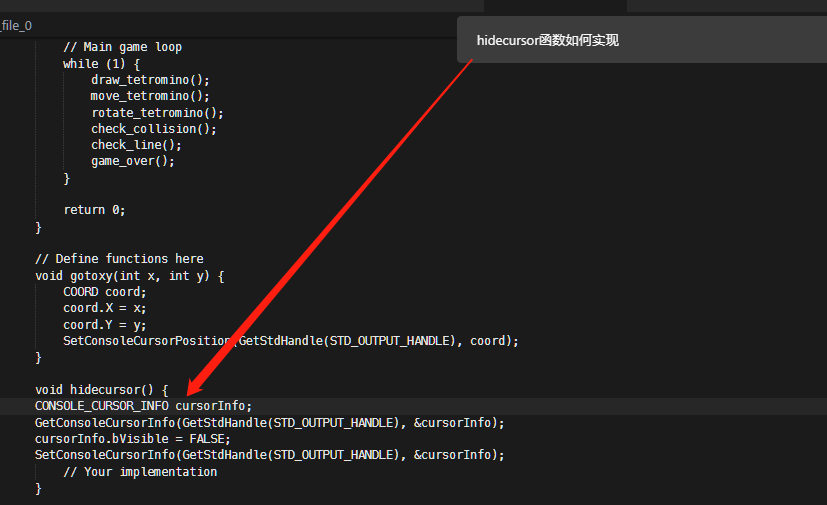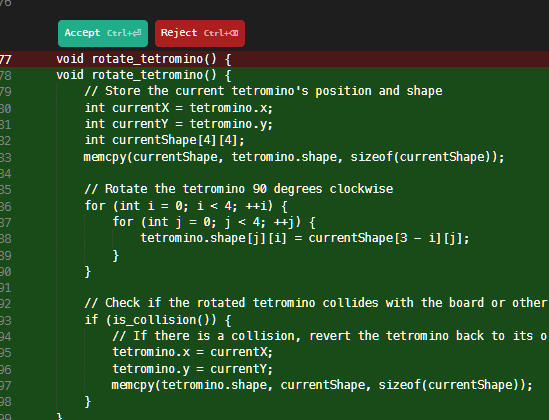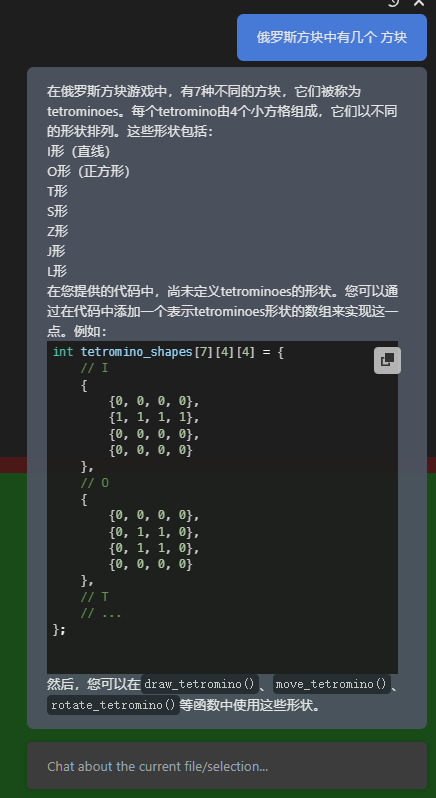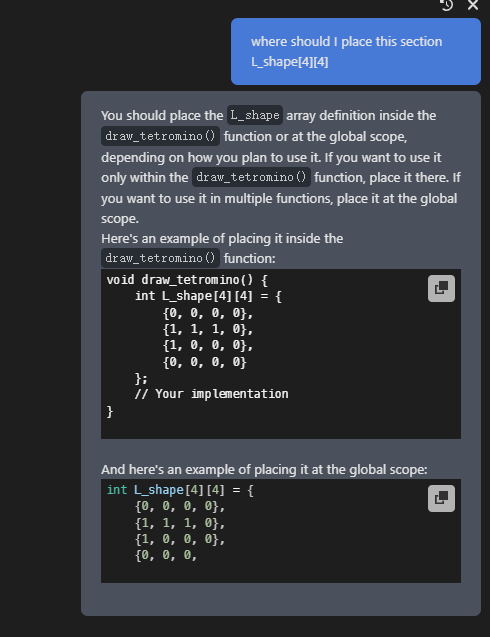# 优化和代码风格

// 用拉格朗日展开求sin函数，JS版本

function lagrangeSin(x, n) {

let sinX = 0;

for (let i = 0; i < n; i++) {

let term = (-1) ** i * x ** (2 * i + 1) / factorial(2 * i + 1);

sinX += term;

}

return sinX;

}

function factorial(n) {

if (n === 0) return 1;

return n * factorial(n – 1);

}

// 用查表法实现sin函数，JS版本，精度为角度制 1度

const sinTable = [];

for (let i = 0; i <= 360; i++) {

sinTable[i] = lagrangeSin((i * Math.PI) / 180, 10);

}

function tableSin(degrees) {

const index = Math.round(degrees) % 360;

return sinTable[index];

}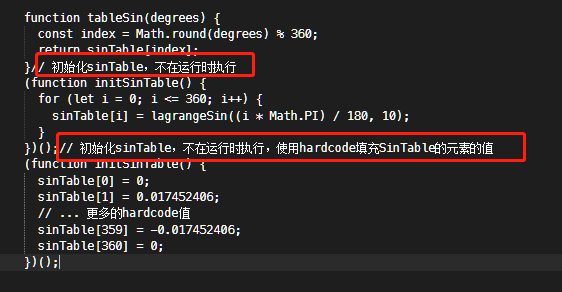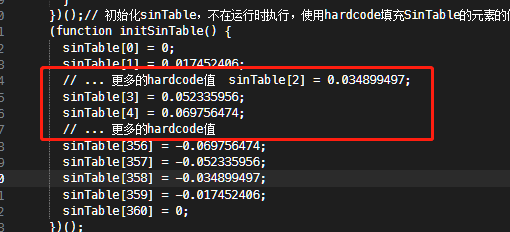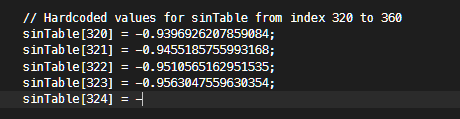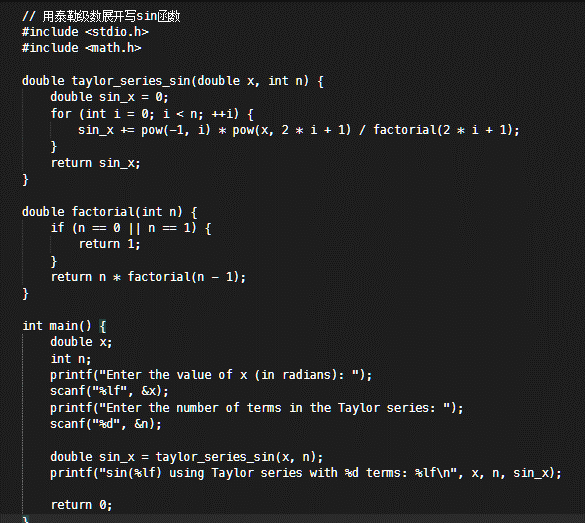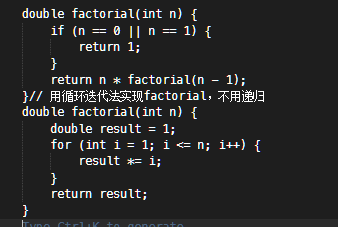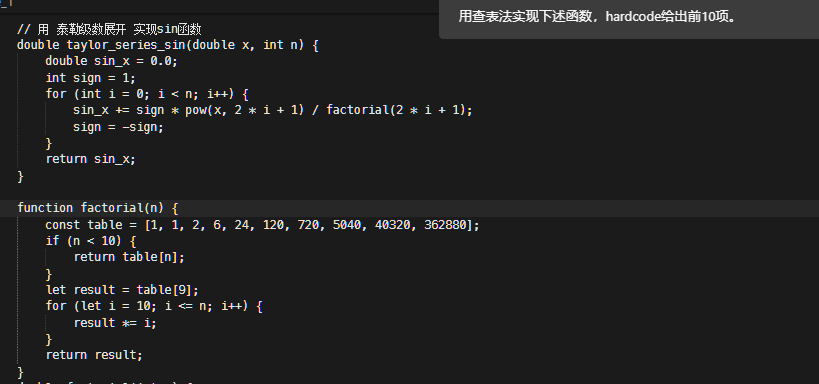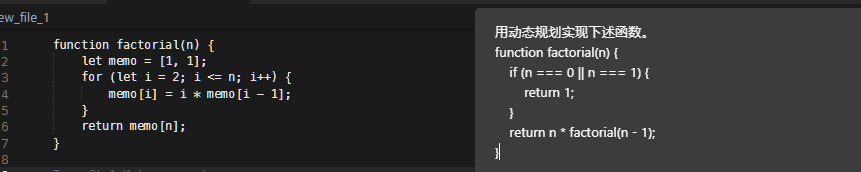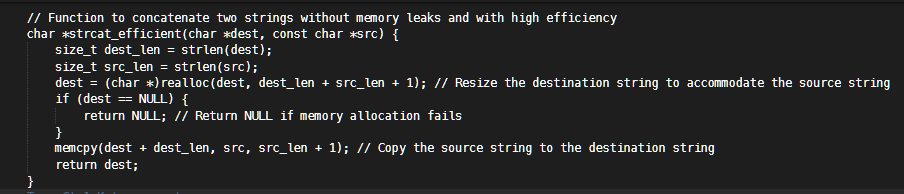// Function to concatenate two strings without memory leaks and with high efficiency

// Returns 0 on success, -1 on failure

// Check if the source string is longer than the allowed limit

// Check if the length of dest is larger than src

# 体会